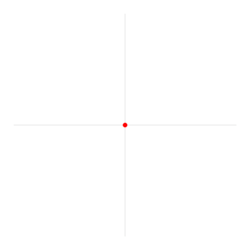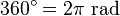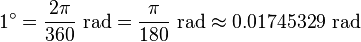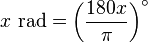Kids Encyclopedia FactsA radian is a unit of angular measure. A revolution of a circle has an angle of 2π radians.

A radian is a unit of measuring angles. It is shown by the symbol "rad" or, less often, c (for circular measure). The radian was once an SI supplementary unit, but was changed to a derived unit in 1995.

## Usage

Most people who do mathematics or physics use radians, rather than degrees, because some kinds of calculations, mostly in trigonometry and calculus, are simpler when using radians rather than degrees. Thus, most calculations related to angular frequency (such as angular velocity) use radians per second.

People who look through a telescope or sniper scope often use milliradians to describe distances as seen through it.

## Conversion$2\pi\mbox{ rad} = 360^\circ$$1 \mbox{ rad} = \frac {360^\circ} {2 \pi} = \frac {180^\circ} {\pi} \approx 57.29577951^\circ$

or:$360^\circ=2\pi\mbox{ rad}$$1^\circ=\frac{2\pi}{360}\mbox{ rad}=\frac{\pi}{180}\mbox{ rad} \approx 0.01745329\mbox{ rad}$

we can also say that:$x \mbox{ rad} = \left(\frac{180x}{\pi}\right)^\circ$.

## Related pagesRadian Facts for Kids. Kiddle Encyclopedia.